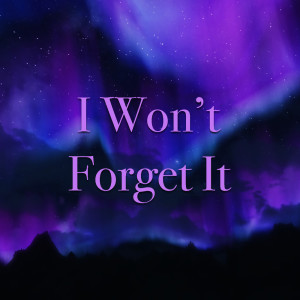# Where The Cash At (Explicit)

15 Jul 2016

## Lirik Where The Cash At (Explicit)

Where The Cash At - Lil Wayne (李尔·韦恩)/Currency/Remmy Ma

You catch my girl legs open

Better smash that

Don't be surprised if she ask

Where the cash at

Where the where the cash at

Where the cash at

Where the where the cash at

Where the cash at

Where the where the cash at

Where the cash at

Don't be surprised if she ask

Where the cash at

Hold on hold on hold on

This song right here is my ni**a

Currency the hot spitter

(That's right)

The very first artist off young

Money cash money

'Bout to hit y'all in the head with

That mothaf**kin' where the cash at

Featuring Remy Martin

And my mothaf**kin' self

Man do what you da li'l daddy

You catch my girl legs open

Better smash that

Don't be surprised if she ask

Where the cash at

Where the where the cash at

Where the cash at

Where the where the cash at

Where the cash at

Where the where the cash at

Where the cash at

Don't be surprised if she ask

Where the cash at

Where the where the cash at

Where the cash at

Where the where the cash at

Where the cash at

Where the where the cash at

Where the cash at

Don't be surprised if she ask

Where the cash at

B**ches come a dime a dozen

I f**k them sisters cousins

It's a revolving dough p**sy

Going p**sy coming

And when it goes to you its coming

Back to me with money

I tell her keep them shoes on

And keep my paper running

I put my mack down just like a

G's supposed to

And if she don't come home

With it then she's sleeping over

I got them creeping over

We do it with the soda

I like them Amery's you can

Have them amerossas

Them niggas down below us

Baby we up and over

I'm trying to get inside that thang

I'm talking large toters

I'm talking large motors

I'm talking burning rubber

That melt in Pirelli odor

I'm hopping out a Lotus

You looking at my b**ch

You wanna holla you can

Holla take her on a trip

But when the deed done

You gotta send her back

Don't be surprised if she ask

Where the cash at

Where the where the cash at

Where the cash at

Where the where the cash at

Where the cash at

Where the where the cash at

Where the cash at

Don't be surprised if she ask

Where the cash at

Where the where the cash at

Where the cash at

Where the where the cash at

Where the cash at

Where the where the cash at

Where the cash at

Don't be surprised if she ask

Where the cash at

I got a b**ch in the back

I got a hoe in the front

I got purp in the dutch

Got purp in the cup

I tell her get your money hoe

Or just stop breathing

'Cause if it ain't about that dough

See a gangster like myself

I get that paper and p**sy

Then there's when I make

That p**sy bring the paper right to me

B**ch you're looking at a real

Pimp that's my old hoe

And they'll tell you no remote

I control hoes

I'm a hoe lord

Give ya that game mamma

If he ain't paying then he

Shouldn't be playin'

I'm saying broke niggas

Only make jokes ni**a

I make more than

I can fit in this quote ni**a

Unquote come sun soak

With me b**ch

Fly you down here and put you

On some south beach sh*t

And if you smile at my b**ch

I make her smile back

Don't be surprised if she ask

Where the cash at

Where the where the cash at

Where the cash at

Where the where the cash at

Where the cash at

Where the where the cash at

Where the cash at

Don't be surprised if she ask

Where the cash at

Where the where the cash at

Where the cash at

Where the where the cash at

Where the cash at

Where the where the cash at

Where the cash at

Don't be surprised if she ask

Where the cash at

See that other chick asked that

She said where the cash at

She said where the paper plastic

Product and where the stash at

With it she said the whip and the

Clip and where's the address at

So that b**ch that be hiding sh*t

In the a** crack

Niggas don't f**kin' call be baby like

I'm related to weezy

Some of them call me aura none

Of them call me easy

Found out I'm f**kin' a man

Then his burners calling me screaming

Chicken wing b**ches I be finding

They just call me Jesus

Yeah I know she got a man

But I'm cool being the other one

'Cause he can't get tight

When he hear I picked another one

I don't fall in love with them cum

And then I'm done with them

Most they get from me is

What p**sy is some bubble gum

But this one dude had me laughing

If its one thing that I can't stand

It's a baggy Magnum

I thought somebody

Through me a camera

The way I caught a flash back

Don't be surprised if she ask

Where the cash at

Where the where the cash at

Where the cash at

Where the where the cash at

Where the cash at

Where the where the cash at

Where the cash at

Don't be surprised if she ask

Where the cash at

Where the where the cash at

Where the cash at

Where the where the cash at

Where the cash at

Where the where the cash at

Where the cash at

Don't be surprised if she ask

Where the cash at